Courses

# Ex 3.5 NCERT Solutions- Pair of Linear Equations in Two Variables Class 10 Notes | EduRev

## Class 10 : Ex 3.5 NCERT Solutions- Pair of Linear Equations in Two Variables Class 10 Notes | EduRev

The document Ex 3.5 NCERT Solutions- Pair of Linear Equations in Two Variables Class 10 Notes | EduRev is a part of the Class 10 Course Mathematics (Maths) Class 10.
All you need of Class 10 at this link: Class 10

Ques 1: Which of the following pairs of linear equations has unique solution, no solution, or infinitely many solutions. In case there is a unique solution, find it by using cross multiplication method.
(i) x - 3y - 3 = 0
3x - 9y - 2 = 0

(ii) 2x + y = 5
3x + 2y = 8

(iii) 3x - 5y = 20
6x - 10y = 40

(iv) x - 3y - 7 = 0
3x - 3y - 15 = 0
Sol: Comparing the equations of each of the given systems with the general system of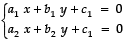We get:
(i) For x - 3y - 3 = 0
3x - 9y - 2 = 0
a1 = 1, b1 = - 3, c1 = - 3
a2 = 3, b2 = - 9, c2 = - 2
We find that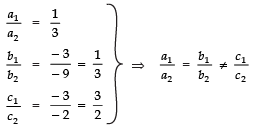∴ The given system has no solution.
(ii) For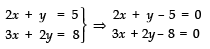a1 = 2, b1 = 1, c1 = - 5
a2 = 3, b2 = 2, c2 = - 8
We find that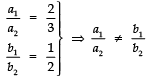∴ The given system has a unique solution.
To solve the equations, we have: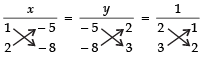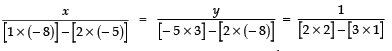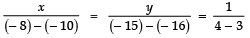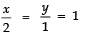⇒ x = 2 and y = 1
(iii) For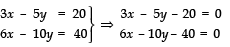a1 = 3, b1 = −5, c1 = − 20
a2 = 6, b2 = − 10, c2 = − 40
Since,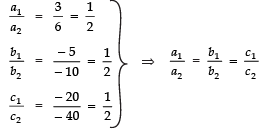∴ The given system of linear equations has infinitely many solutions.
(iv) For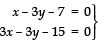a1 = 1, b1 = − 3, c1 = − 7
a2 = 3, b1 = − 3, c2 = − 15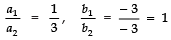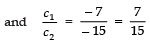Since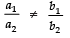∴ The given system has a unique solution.
Now, to use cross multiplication method, we have: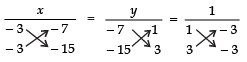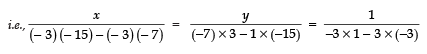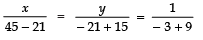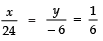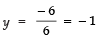Thus, x = 4 and y = -1

Ques 2: (i) For which values of a and b does the following pair of linear equations have an infinite number of solutions?
2x + 3y = 7
(a - b) x + (a + b) y = 3a + b - 2
(ii) For which value of k will the following pair of linear equations have no solution?
3x + y = 1
(2k - 1) x + (k - 1) y = 2k + 1
Sol: (i) Comparing
2x + 3y = 7
(a - b) x + (a + b) y = (3a + b - 2)
with A1 x + B1 y = C1
A2 x + B2 y = C2
we get:
A1 = 2, B1 = 3, C1 = 7
A2 = (a - b), B2 = (a + b), C2 = (3a + b - 2)
For an infinite number of solutions,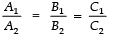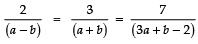From the first two equations, we get: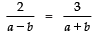⇒ 2 (a + b)=3 (a − b)
⇒ 2a + 2b =3a − 3b
⇒ 2a − 3a + 2b + 3b = 0
⇒ − a + 5b = 0 ⇒ a − 5b = 0    ...(1)
From the last two equations,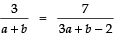⇒ 3 (3a + b − 2) = 7 (a + b)
⇒ 9a + 3b − 6=7a + 7b
⇒ 9a − 7a + 3b − 7b − 6 = 0
⇒ 2a − 4b = 6
⇒ a − 2b = 3    ...(2)
Now, to solve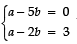by cross multiplication method:
A1 = 1, B1 = - 5, C1 = 0
A2 = 1, B2 = - 2, C2 = 0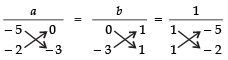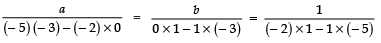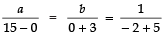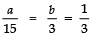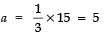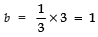Thus, a = 5 and b = 1
(ii) For no solution, we have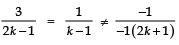Let us consider,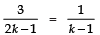⇒ 3 (k − 1) = 1 (2k − 1)
⇒ 3k − 3 = 2k − 1
⇒ 3k − 2k = −1 +
⇒ k = 2
∴ For k = 2, the given pair of linear equations will have no solution.

Ques 3: Solve the following pair of linear equations by the substitution and cross-multiplication methods:
8x + 5y = 9
3x + 2y = 4
Sol: Method - 1 [using Substitution Method]
8x + 5y = 9     ...(1)
3x + 2y = 4     ...(2)
From (2),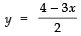Substituting this value of y in (1), we have: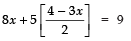⇒ 2 × 8x + 5 × x - 5 × 3x = 9 × 2
⇒ 16x + 20 - 15x = 18
⇒ x + 20 - 18 = 0
⇒ x + 2 = 0 ⇒ x = - 2
Now, putting x = (- 2) in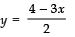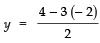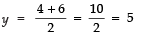Thus, x = - 2 and y = 5
Method - II [Cross Multiplication Method]
Comparing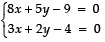with general pair of equations,
a1 = 8, b1 = 5, c1 = - 9
a2 = 3, b= 2, c2 = - 4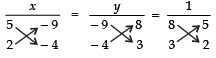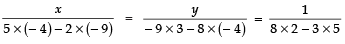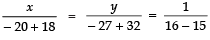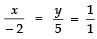⇒ x = 1 × - 2 = - 2 and y = 1 × 5 = 5
Thus x = - 2 and y = 5

Ques 4: Form the pair of linear equations in the following problems and find their solutions (if they exist) by any algebraic method:
(i) A part of monthly hostel charges is fixed and the remaining depends on the number of days one has taken food in the mess. When a student A takes food for 20 days she has to pay ₹ 1000 as hostel charges whereas a student B, who takes food for 26 days, pays ₹ 1180 as hostel charges. Find the fixed charges and the cost of food per day.
(ii) A fraction becomes 1/3 when 1 is subtracted from the numerator and it becomes 1/4 when 8 is added to its denominator. Find the fraction.
(iii) Yash scored 40 marks in a test, getting 3 marks for each right answer and losing 1 mark for each wrong answer. Had 4 marks been awarded for each correct answer and 2 marks been deducted for each incorrect answer, then Yash would have scored 50 marks. How many questions were there in the test?
(iv) Places A and B are 100 km apart on a highway. One car starts from A and another from B at the same time. If the cars travel in the same direction at different speeds, they meet in 5 hours. If they travel towards each other, they meet in 1 hour. What are the speeds of the two cars?
(v) The area of a rectangle gets reduced by 9 square units, if its length is reduced by 5 units and breadth is increased by 3 units. If we increase the length by 3 units and the breadth by 2 units, the area increases by 67 square units. Find the dimensions of the rectangle.
Sol: (i) Let the fixed charges = ₹ x
And charges of food per day = ₹ y
For student A:
Number of days = 20
∴ Cost of food for 20 days = ₹ 20y
According to the problem,
x + 20y = 1000     ...(1)
For student B:
Number of days = 26
∴ Cost of food for 26 days = ₹ 26y
According to the problem:
x + 26y = 1180   ...(2)
Now, we have the system of linear equations: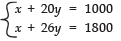We have: a1 = 1, b1 = 20, c1 = - 1000
a2 = 1, b2 = 26, c2 = - 1800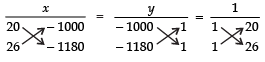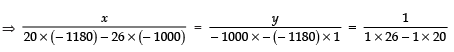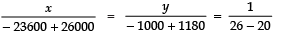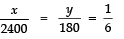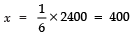Thus,  x = 400 and y = 30
∴Fixed charges = ₹ 400 and cost of food per day = ₹ 30
(ii) Let the numerator = x
And the denominator = y
∴  Fraction = x/y
Case-I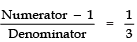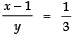⇒ 3 (x − 1) = y
⇒ 3x − 3 = y
⇒ 3x − y − 3 = 0     ..(1)
Case-II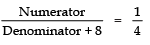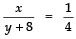⇒ 4 x = y + 8
⇒ 4x − y − 8 = 0     ...(2)
Comparing (1) and with the general pair of linear equations,
a1 = 3, b1 = - 1, c1 = - 3
a2 = 4, b2 = - 1, c2 = - 8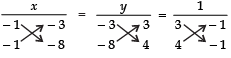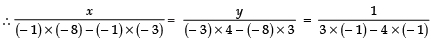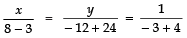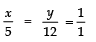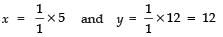Thus, x = 5 and y = 12
∴ Fraction  = 5/12
(iii) Let the number of correct answers = x
And the number of wrong answers = y
Case-I
Marks for all correct answer (3 × x) = 3x
Marks for all wrong answer (1 × y) = y
∴ According to the conditions:
3x - y = 40 ...(1)
Case-II
Marks for all correct answers (4 × x) = 4x
Marks for all wrong answers (2 × y) = 2y
∴ According to the condition:
4x - 2y = 50
⇒ 2x - y = 25 ...(2)
From (1) and (2) we have:
a1 = 3, b1 = - 1, c1 = - 40
a2 = 2, b2 = - 1, c2 = - 25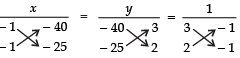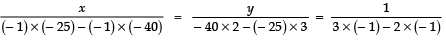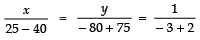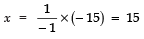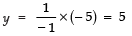∴  x = 15 and y = 5
Now, total questions = [Number of correct answers] + [Number of wrong answers]
= 15 + 5
= 20
Thus, required number of questions = 20.
(iv) Let the speed of car-I be x km/hr
And the speed of car-II be y km/hr
Case-I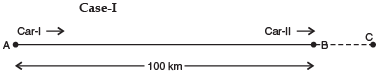Distance travelled by car-I = AC
⇒ speed × time = 5 × x km
AC = 5x
Distance travelled by car-II = BC = 5y
Since AB = AC - BC
100 = 5x - 5y
⇒ 5x - 5y - 100 = 0
⇒ x - y - 20 = 0    ...(1)
Case-II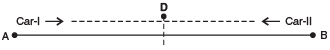Distance travelled by car-I in 1 hour = AD
∴ AD = 1 × x = x
Distance travelled by car-II in 1 hour = BD
∴ BD = 1 × y = y
Now AB = AD + DB
⇒ 100 = x + y
⇒ x + y = 100     ...(2)
Using cross-multiplication,
x - y - 20 = 0
x + y - 100 = 0
a1 = 1, b1 = - 1, c1 = - 20
a2 = 1, b2 = 1, c2 = - 100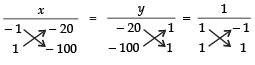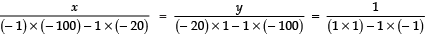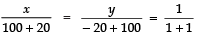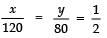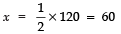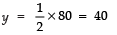Thus, speed of car-I = 60 km/hr
speed of car-II = 40 km/hr.
(v) Let the length of the rectangle = x units
And the breadth of the rectangle = y units.
∴ Area of the rectangle = x × y = xy
Condition-I:
(Length - 5) × (Breadth + 3) = Area - 9
⇒ (x - 5) (y + 3) = xy - 9
⇒ xy + 3x - 5y - 15 = xy - 9
⇒ xy + 3x - 5y - 15 - xy + 9 = 0
⇒ 3x - 5y - 6 = 0 ...(1)
Condition-II:
(Length + 3) × (Breadth + 2) = Area + 67
⇒ (x + 3) (y + 2) = xy + 67
⇒ xy + 2x + 3y + 6 = xy + 67
⇒ xy + 2x + 3y + 6 - xy - 67 = 0
⇒ 2x + 3y - 61 = 0      ...(2)
Now, using cross multiplication method for (1) and (2), we have:
a1 = 3, b1 = - 5, c1 = - 6
a2 = 2, b2 = 3, c2 = - 61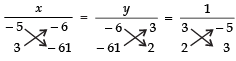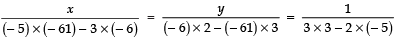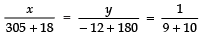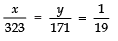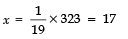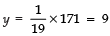Thus, length of the rectangle = 17 units
And breadth of the rectangle = 9 units.
Equations Reducible to Pair of Linear Equations in Two Variables

Offer running on EduRev: Apply code STAYHOME200 to get INR 200 off on our premium plan EduRev Infinity!

## Mathematics (Maths) Class 10

178 videos|268 docs|103 tests

,

,

,

,

,

,

,

,

,

,

,

,

,

,

,

,

,

,

,

,

,

;